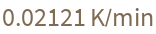Function Repository Resource:

ColdWaterSurvivalTime

Compute the approximate survival time of a human in cold water

Contributed by: Claudio Lobo Chaib Filho
 ResourceFunction["ColdWaterSurvivalTime"][temp] estimates the survival time in cold water for a temperature temp. ResourceFunction["ColdWaterSurvivalTime"][temp,prop] gives the property prop.

Details and Options

ResourceFunction["ColdWaterSurvivalTime"] is useful to inform how long a person can survive hypothermia.
Any temperature quantity is accepted by the function. If the argument is not a Quantity, it is interpreted as "DegreesCelsius".
The temperature values temp accepted by the function must be in the temperature range 0 to 20 degrees Celsius.
Available properties include:
 "SurvivalTime" time a human can survive in cold water of the specified temperature "MetabolicHeat" heat generated by the body in the cold water "CoolingRate" body's cooling rate "Plot" plot of the curve of survival time versus water temperature "PropertyAssociation" all results as an Association
ResourceFunction["ColdWaterSurvivalTime"][temp] is equivalent to ResourceFunction["ColdWaterSurvivalTime"][temp,"SurvivalTime"].
The "Plot" property shows the survival time curve. The x axis is the water temperature and y axis is the survival time. The input temp is displayed as a red point.
ColdWaterSurvivalTime accepts the options:
 "EnergyUnit" "Calories" unit of the result heat ("Kilojoules", "Watts", …) "TimeUnit" "Minutes" unit of time in the results

Examples

Basic Examples

Get the survival time in cold water at a specific temperature:

 In:=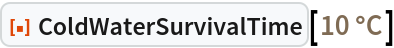Out=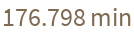Scope

The function interprets any unit type of degrees for temperature:

 In:=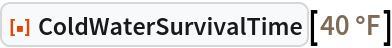Out=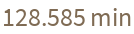The function used with one argument is similar to the function with two arguments where the second is "SurvivalTime":

 In:=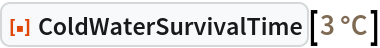Out=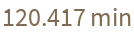In:=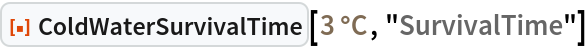Out=If a Quantity is not specified, it is interpreted in "DegreesCelsius":

 In:=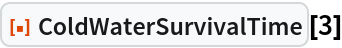Out=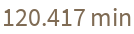Obtain the heat generated by the body in water at the specified temperature:

 In:=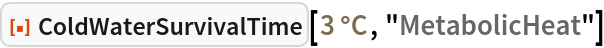Out=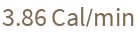Obtain the body’s cooling rate for a specific temperature:

 In:=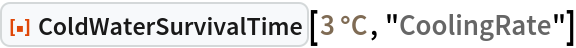Out=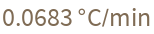Use "Plot" to obtain the survival time curve (y axis) for different water temperatures (x axis):

 In:=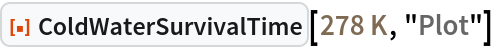Out=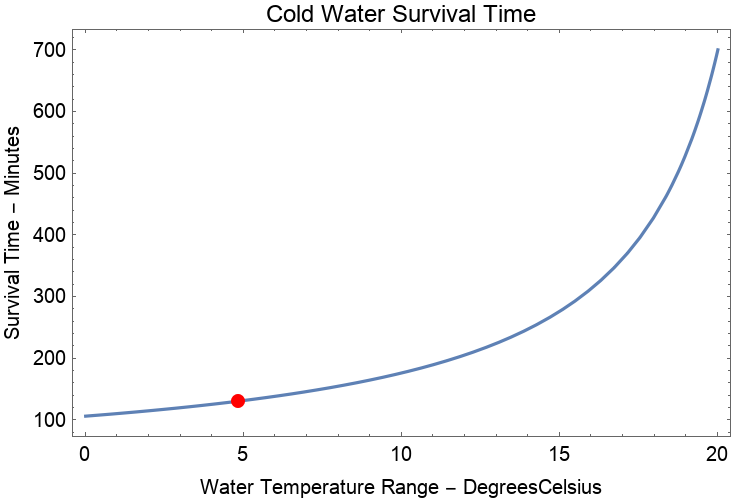Use "PropertyAssociation" to get all the information simultaneously for a specific temperature. Note that the result is given in the same temperature units as the input:

 In:=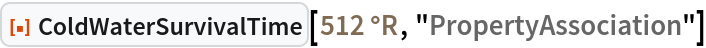Out=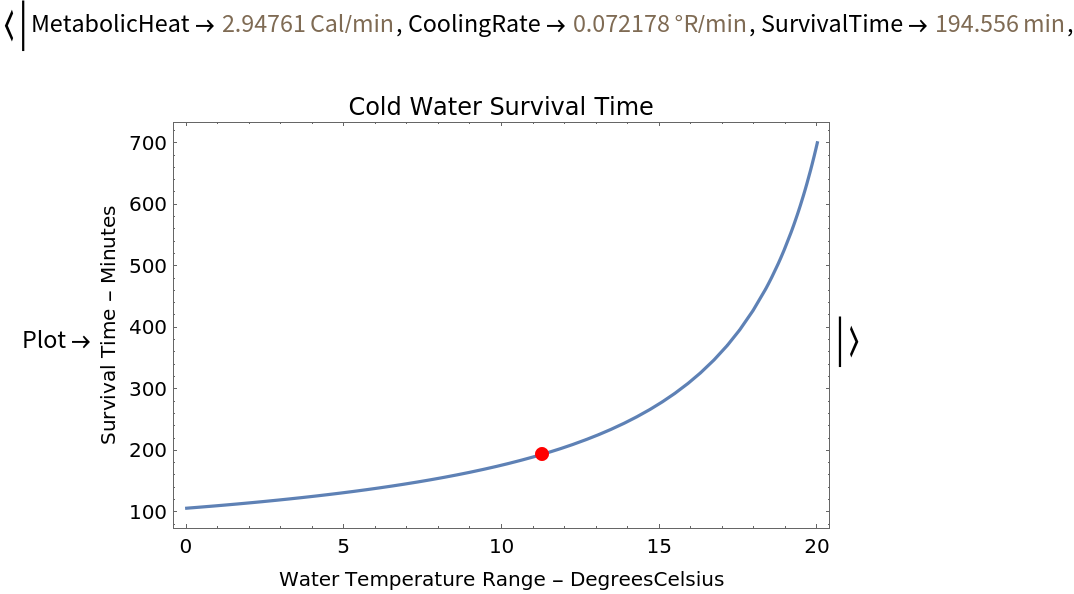Options

Get information using another unit of heat with the option "EnergyUnit":

 In:=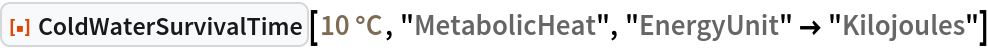Out=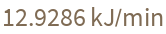Choose another unit of time with the "TimeUnit" option:

 In:=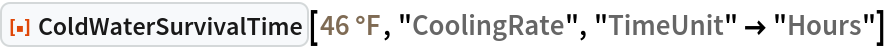Out=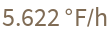Change both the unit of energy and the time. The plot and the result use the chosen time unit and a given requested energy unit:

 In:=Out=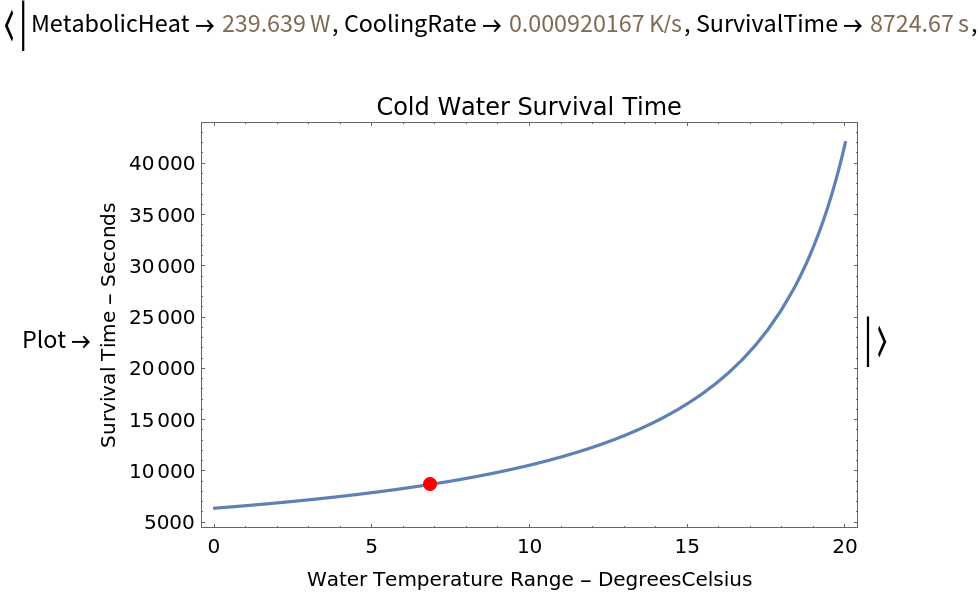Possible Issues

Note that even if the temperature unit is not degrees Celsius, the x axis of the graph remains in that unit:

 In:=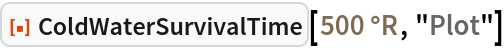Out=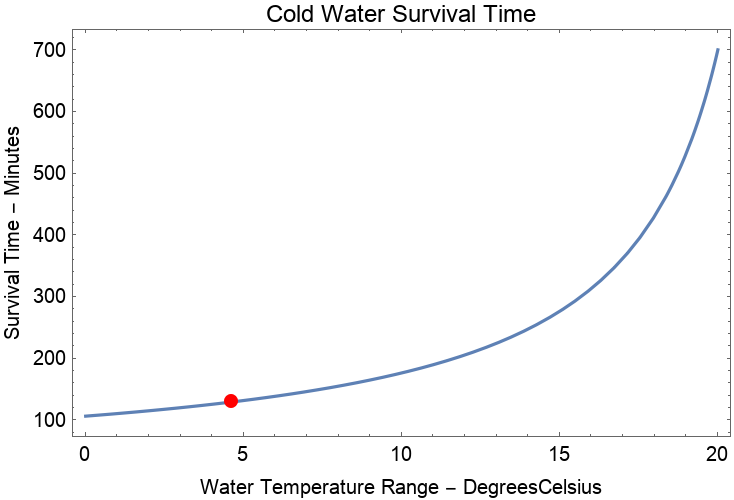If the required property does not contain information about the energy, the "EnergyUnit" option does not influence the output:

 In:=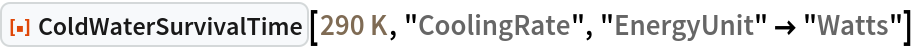Out=